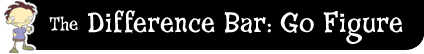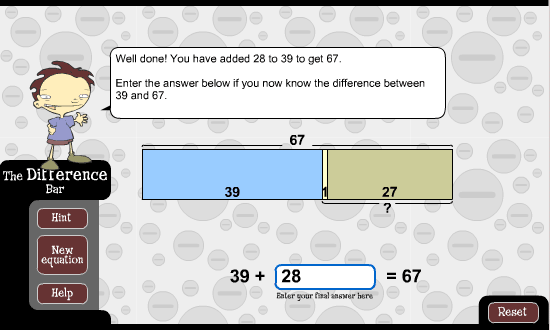﻿ The difference bar: go figure: round to the nearest 10Round to the nearest 10

The idea behind this strategy is to work out the difference by rounding the first number in the equation up to its nearest 10. Then add on the remainder to give you the final difference.

For example, let's look at the difference between 66 and 75. This is the same as 66 + ? = 75. First, round up 66 by 4 to make 70. Next, add 5 to get to 75. Then, work out that 4 plus 5 has been added, totalling 9. So, the difference between 66 and 75 is 9.I could also use this strategy for harder differences like 39 and 67. This is the same as 39 + ? = 67. First, add 1 to round 39 up to 40. Then, add 27 more to make 67. In total I've added 1 plus 27 making 28. So, the difference between 39 and 67 is 28.Let's use this strategy to work out the difference between 26 and 73. This is the same as 26 + ? = 73. First, round 26 to the nearest 10, which is 30. Next, add on the remaining difference between 30 and 73.

Did you get 4 for the amount you rounded? Did you then add 43? Add these numbers together to get the final difference.

Did you get 47?

Now try to work out the difference between 13 and 22. Remember, this is the same as 13 + ? = 22.